# How to Automatically Merge Blank Cells Above/Left in Excel?

Merging is one of the most complicated processes in Excel. If we merge the cells incorrectly, data may be lost and cannot be recovered. We can use the process mentioned in this article to do it more carefully, which only considers empty cells. This tutorial will help you understand how we can automatically merge blank cells above or left in Excel. Combining multiple cells together is known as merging.

## Automatically Merge Blank Cells Above

Here we will insert a VBA module and then run it to complete our task. Let us see a straightforward process to understand how we can automatically merge blank cells above in Excel using the vba code.

Step 1

Consider an Excel sheet with data similar to the one shown in the image below.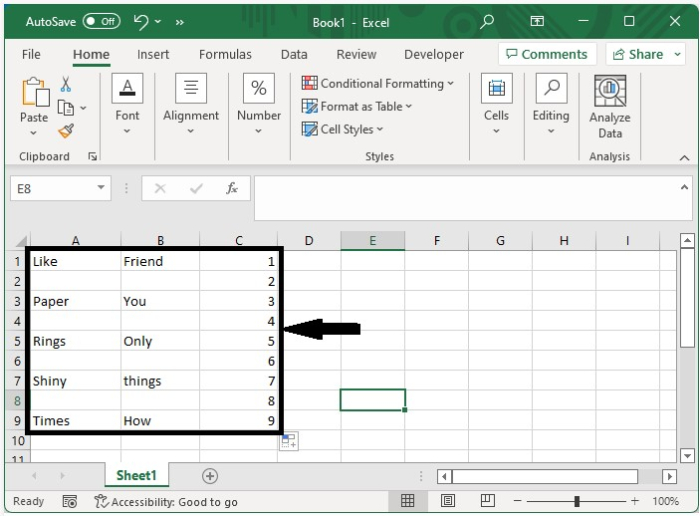To open the VBA application, right-click on the sheet name and select View Code, then click on the inset and select Module, and type Program 1 into the text box as shown in the below image.

### Example 1

Sub MergeCells()
'Update By Nirmal
Dim xRg As Range
Dim xCell As Range
On Error Resume Next
Set xRg = Application.InputBox("Select a range:", "Choose the Range", xAddress, , , , , 8)
If xRg Is Nothing Then Exit Sub
For Each xCell In xRg
If xCell.Value = "" Then
Range(xCell, xCell.Offset(-1, 0)).Merge
End If
Next
End Sub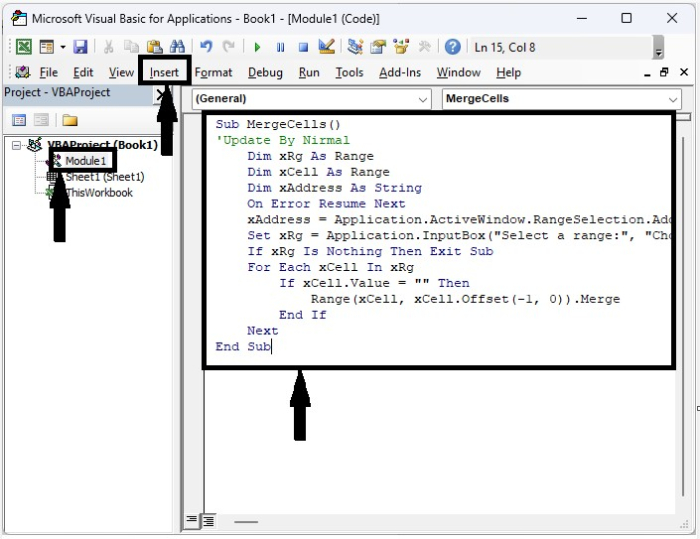Step 2

Now click on F5 to run the VBA code, then in the pop-up, select the range you want to merge, and click on OK.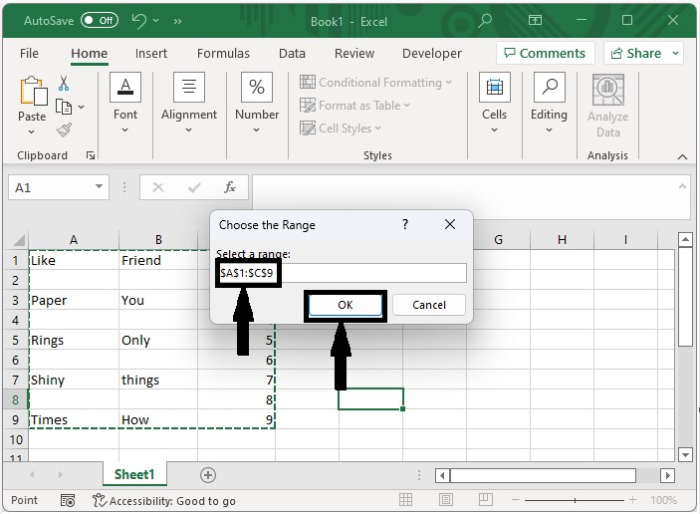Our final output will look like the below image.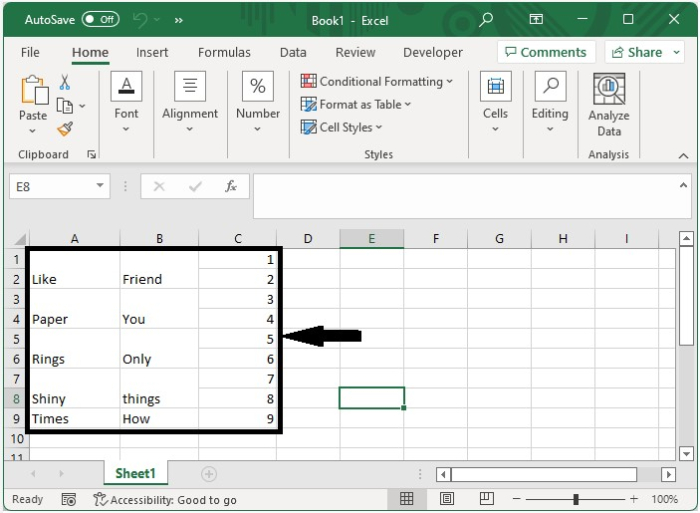If you only want to allow mering for one of the cells above, use Program 2 in the VBA application.

### Example 2

Sub mergeblankswithabove()
'Updated By Nirmal
Dim I As Long
Dim xRow As Long
Dim xRg As Range
Dim xCell As Range
On Error Resume Next
Set xRg = Application.InputBox("Select a range (single column):", "Choose the Range", xAddress, , , , , 8)
If xRg Is Nothing Then Exit Sub
If xRg.Columns.Count > 1 Then
MsgBox "Only work for single column", , "Range selected"
Exit Sub
End If
xRow = xRg.Rows.Count
Set xRg = xRg(xRow)
For I = xRow To 1 Step -1
Set xCell = xRg.Offset(I - xRow, 0)
If xCell.Value = "" Then Range(xCell, xCell.Offset(-1, 0)).Merge
Next
End Sub


## Automatically Merge Blank Cells Left

Here we will insert the VBA module and then run it to complete our task. Let us see a straightforward process to understand how we can automatically merge blank cells. left in Excel using the VBA code.

Step 1

Consider an Excel sheet with data similar to the one shown in the image below.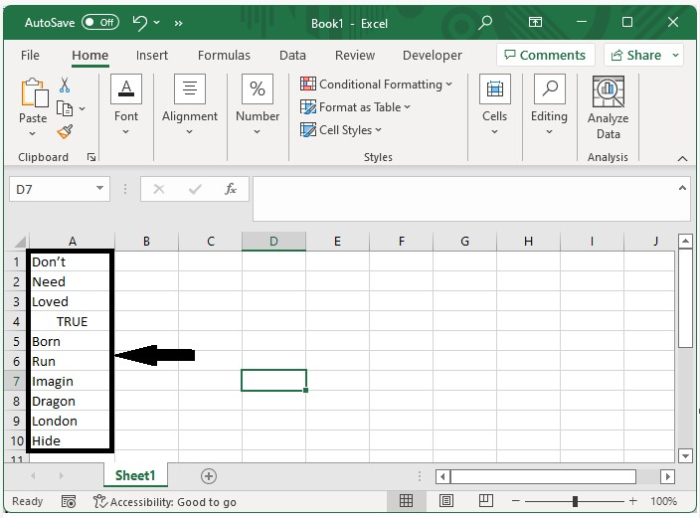To open the VBA application, right-click on the sheet name and select View Code, then click on the inset and select Module, and type Program 3 into the text box as shown in the below image.

### Example 3

Sub mergeblankswithleft()
'Update by Nirmal
Dim xRg As Range
Dim xCell As Range
On Error Resume Next
Set xRg = Application.InputBox("Select a range:", "Range to merge", xAddress, , , , , 8)
If xRg Is Nothing Then Exit Sub
For Each xCell In xRg
If xCell.Value = "" Then Range(xCell, xCell.Offset(0, -1)).Merge
Next
End Sub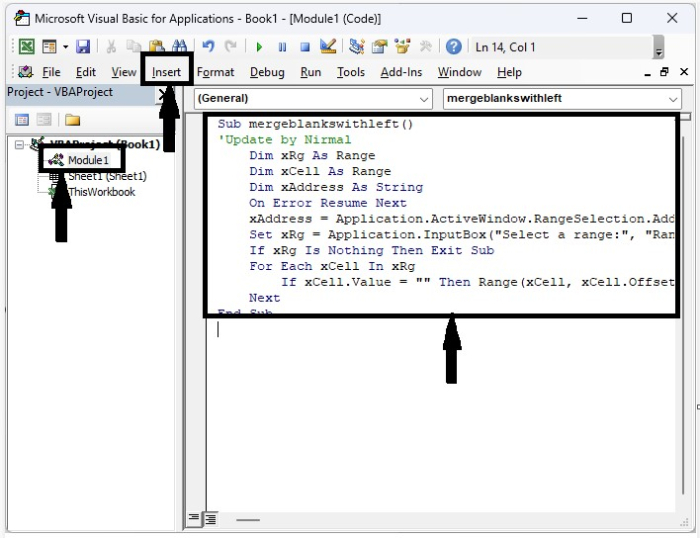Step 2

Now click on F5 to run the vba code, then in the pop-up, select the range you want to merge, and click on "OK." Our final output will look like the below image.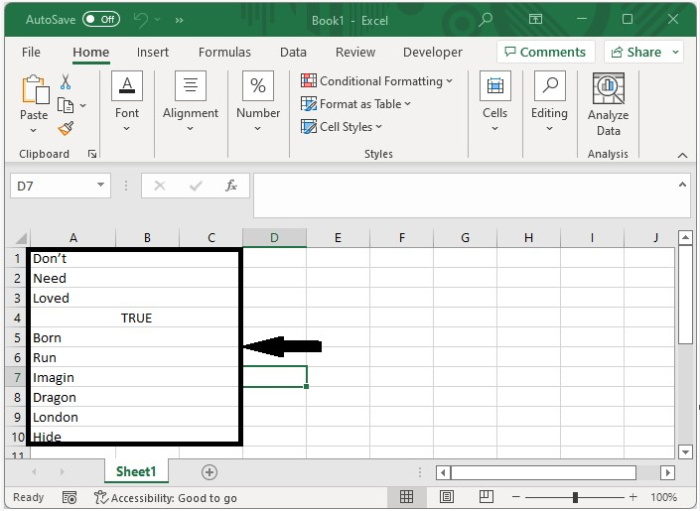## Conclusion

In this tutorial, we used a simple example to demonstrate how we can automatically merge blank cells in Excel.

Updated on: 10-Jan-2023

1K+ Views